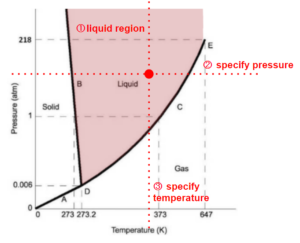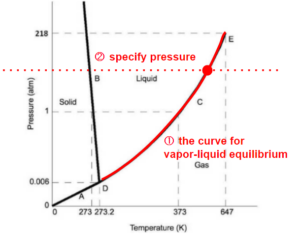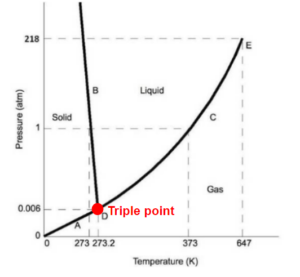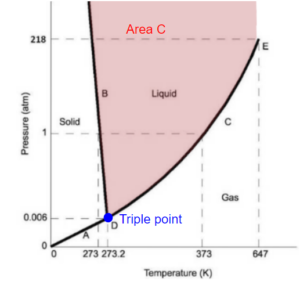# 26 Gibb’s Phase Rule

Learning Objectives

By the end of this section, you should be able to:

Calculate degrees of freedom using Gibb’s Phase Rule

Gibb’s phase rule is used to determine the number of intensive variables required to fully specify the thermodynamic properties of a system (thermodynamic degrees of freedom).

Once this number of intensive variables is specified, we can find all the thermodynamic properties of the substance (eg. other intensive variables such as pressure, temperature, enthalpy, internal energy, etc.) at that state using previous experimental data from a table or chart.

 $D\!F=2+c-\pi-r$

$D\!F$ = degrees of freedom (number of intensive variables that needs to be specified)

$c$ = number of independent chemical species

$\pi$ = number of phases in a system at equilibrium

$r$ = number of independent equilibrium chemical reactions among the species

One way of understanding this equation is thinking that we want to find a point (0 dimension) on the phase diagram, as this represents a single set of thermodynamic properties. If we have a single component system, the phase diagram is similar to the ones we have seen so far in this lecture. Specifying one intensive property reduces the dimension by 1. Adding a component adds another axis to the graph (to represent the composition of the mixture), which adds one dimension. Each equilibrium provides an equation that relates the composition of substances in the system, which allows us to secure a position on the composition axis.

For example, if we have a pure substance in the system and we know that the state is in the liquid region(2 dimension), specifying pressure ensures the point of the state we are looking for is on a horizontal line (1 dimension). If we then also specify the temperature, it ensures the point is on a vertical line. There is only one point that satisfies both requirements of the temperature and pressure. We have reduced the dimension to 0.In another example, if we know the state is on one of the lines indicating state transitions (eg. boiling – being vapour-liquid equilibrium), we start at 1 dimension, then we just need to specify one of the x, y coordinates (temperature or pressure) to reduce the position of the state to a point.Triple point is already a point (0 dimension). No other variables need to be specified.Exercise: Gibb’s Phase Rule

In a system containing pure water, how many degrees of freedom are there in the system at:

a) somewhere in the liquid phase shown by area C.

b) The triple point### Solution

Because we only have water in the system, $c=1$, and there is no equilibrium chemical reaction in the system (as there is nothing to react with and no reaction specified), so $r=0$.

a) At area C, we only have a liquid phase, so $\pi=1$

$D\!F=2+c-\pi-r=2+1-1-0=2$

This area represents pure water as a liquid. To fix this and know its thermodynamic properties we would need to know 2 intensive variables, say temperature and pressure. Knowing these two then rather than having an area, we would have a specific point.

b) At the triple point, pure vapour, liquid, and solid water exist at the same time, so $\pi=3$

$D\!F=2+c-\pi-r=2+1-3-0=0$

Specifying the water is at its triple point is equivalent to specifying the pressure and temperature, because the triple point only exists at a certain set of pressure and temperature.